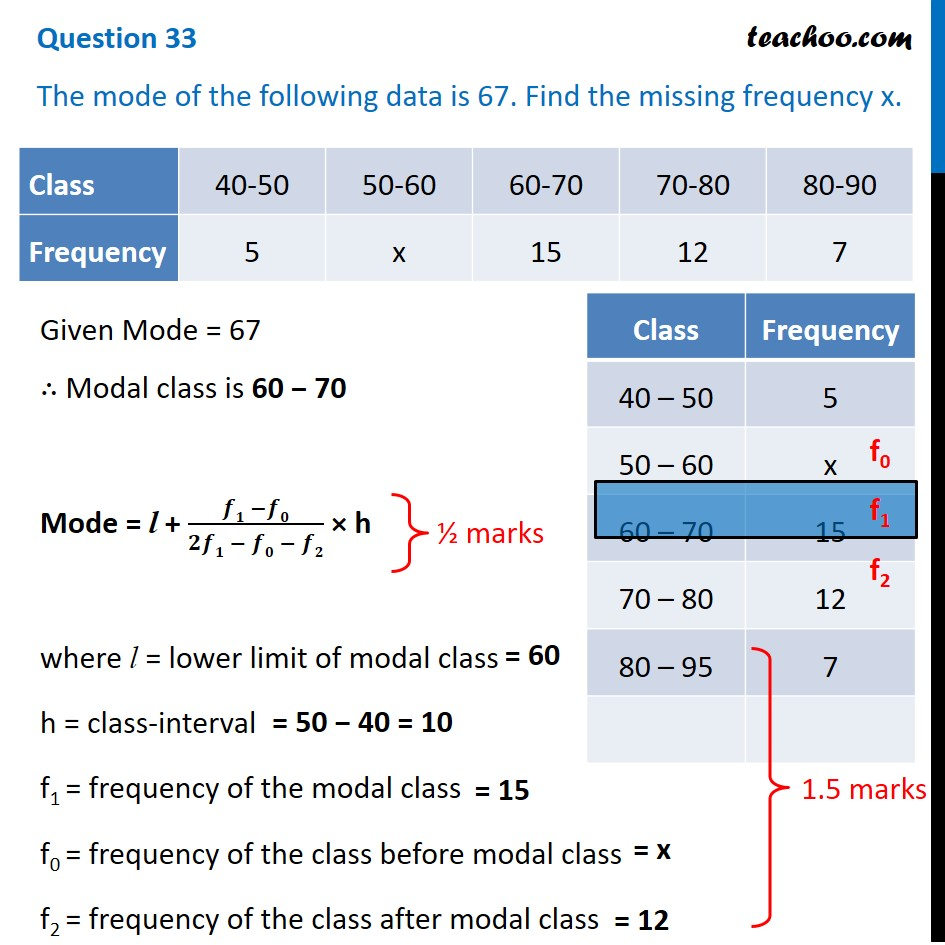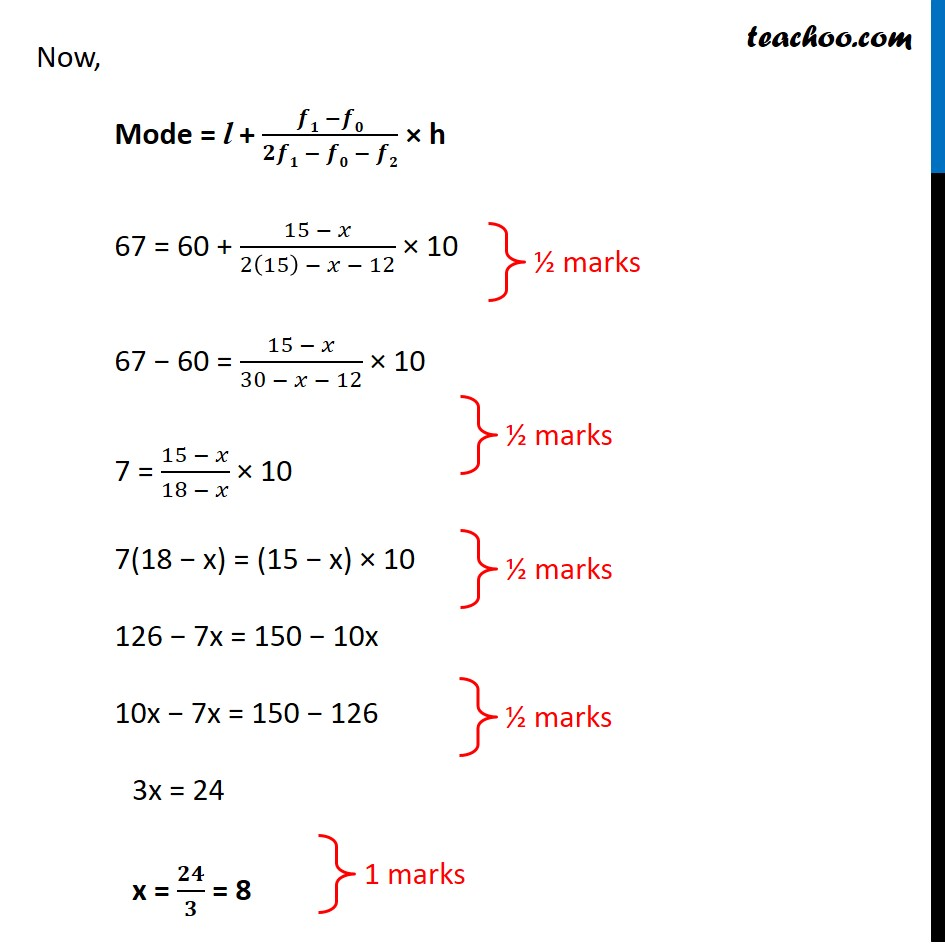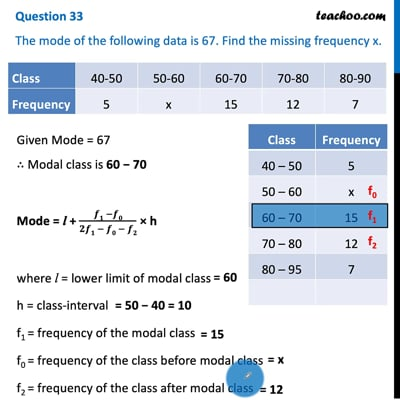CBSE Class 10 Sample Paper for 2021 Boards - Maths Standard

Class 10
Solutions of Sample Papers for Class 10 Boards

## The mode of the following data is 67. Find the missing frequency x.This video is only available for Teachoo black users

Learn in your speed, with individual attention - Teachoo Maths 1-on-1 Class

### Transcript

Question 33 The mode of the following data is 67. Find the missing frequency x.Given Mode = 67 ∴ Modal class is 60 − 70 Mode = l + (𝒇𝟏 −𝒇𝟎)/(𝟐𝒇𝟏 − 𝒇𝟎 − 𝒇𝟐) × h where l = lower limit of modal class h = class-interval f1 = frequency of the modal class f0 = frequency of the class before modal class f2 = frequency of the class after modal class Now, Mode = l + (𝒇𝟏 −𝒇𝟎)/(𝟐𝒇𝟏 − 𝒇𝟎 − 𝒇𝟐) × h 67 = 60 + (15 − 𝑥)/(2(15) − 𝑥 − 12) × 10 67 − 60 = (15 − 𝑥)/(30 − 𝑥 − 12) × 10 7 = (15 − 𝑥)/(18 − 𝑥) × 10 7(18 − x) = (15 − x) × 10 126 − 7x = 150 − 10x 10x − 7x = 150 − 126 3x = 24 x = 𝟐𝟒/𝟑 = 8